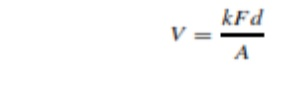Home | | Measurements and Instrumentation | Piezoelectric Transducers

# Piezoelectric Transducers

Piezoelectric transducers produce an output voltage when a force is applied to them. They are frequently used as ultrasonic receivers and also as displacement transducers, particularly as part of devices measuring acceleration, force and pressure.

Piezoelectric Transducers

Piezoelectric transducers produce an output voltage when a force is applied to them. They are frequently used as ultrasonic receivers and also as displacement transducers, particularly as part of devices measuring acceleration, force and pressure. In ultra- sonic receivers, the sinusoidal amplitude variations in the ultrasound wave received are translated into sinusoidal changes in the amplitude of the force applied to the piezoelectric transducer. In a similar way, the translational movement in a displacement transducer is caused by mechanical means to apply a force to the piezoelectric transducer. Piezoelectric transducers are made from piezoelectric materials. These have an asymmetrical lattice of molecules that distorts when a mechanical force is applied to it. This distortion causes a reorientation of electric charges within the material, resulting in a relative displacement of positive and negative charges. The charge displacement induces surface charges on the material of opposite polarity between the two sides. By implanting electrodes into the surface of the material, these surface charges can be measured as an output voltage. For a rectangular block of material, the induced voltage is given by:

V = kFd/AWhere F is the applied force in g, A is the area of the material in mm, d is the thickness of the material and k is the piezoelectric constant. The polarity of the induced voltage depends on whether the material is compressed or stretched.

Where F is the applied force in g, A is the area of the material in mm, d is the thickness of the material and k is the piezoelectric constant. The polarity of the induced voltage depends on whether the material is compressed or stretched.

Materials exhibiting piezoelectric behaviour include natural ones such as quartz, synthetic ones such as lithium sulphate and ferroelectric ceramics such as barium titanate. The piezoelectric constant varies widely between different materials. Typical values of k are 2.3 for quartz and 140 for barium titanate. Applying equation (13.1) for a force of 1 g applied to a crystal of area 100 mm2 and thickness 1 mm gives an output of 23 µV for quartz and 1.4 mV for barium titanate.

The piezoelectric principle is invertible, and therefore distortion in a piezoelectric material can be caused by applying a voltage to it. This is commonly used in ultrasonic transmitters, where the application of a sinusoidal voltage at a frequency in the ultra- sound range causes a sinusoidal variation in the thickness of the material and results in a sound wave being emitted at the chosen frequency. This is considered further in the section below on ultrasonic transducers.

Study Material, Lecturing Notes, Assignment, Reference, Wiki description explanation, brief detail
Measurements and Instrumentation : Transducers and Data Acquisition Systems : Piezoelectric Transducers |